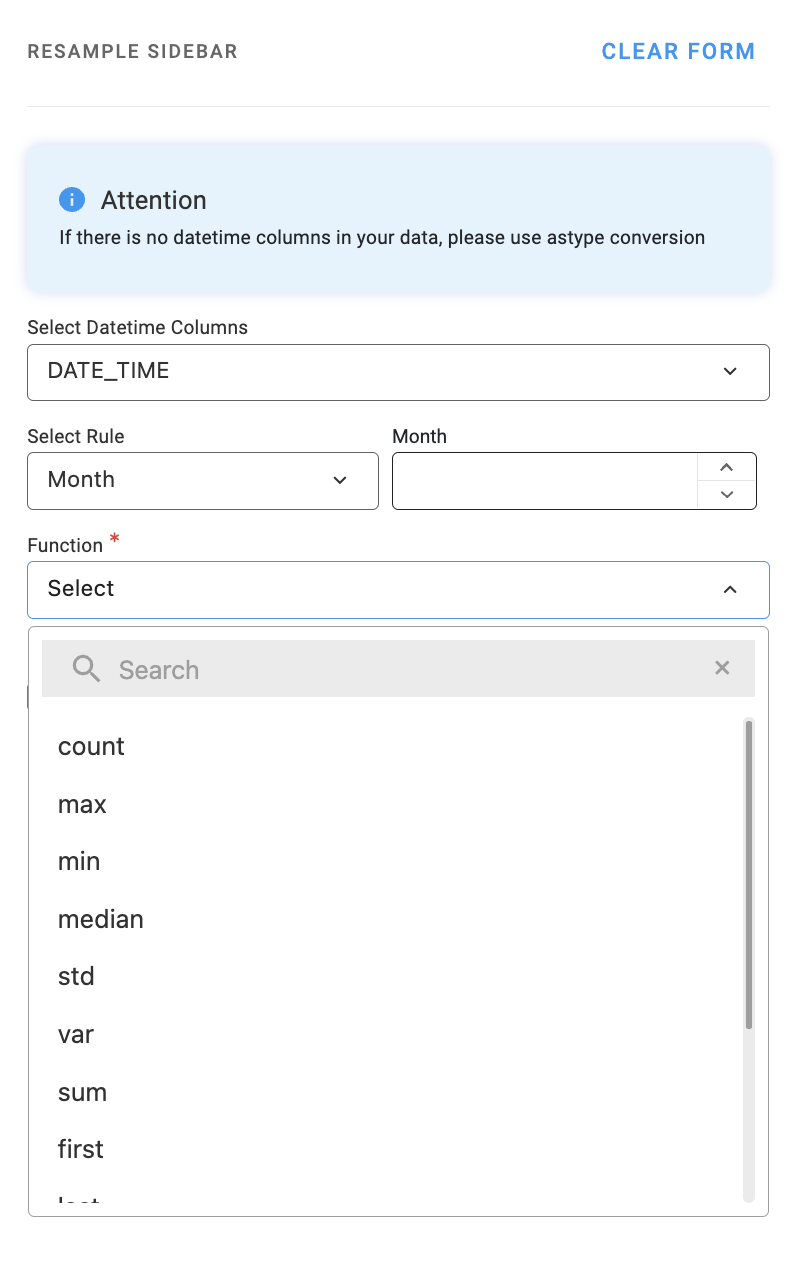# ResampleThe resample function is used to change the frequency of time-series data by aggregating or downsampling the data based on a specified rule. This is particularly useful when working with time-series data where you want to analyze the data at a different frequency, such as daily, weekly, or monthly.The selection rule, or resampling frequency, is specified using a string, such as daily, weekly, monthly, etc. Once the data is resampled, you can apply various aggregation functions to summarize the data at the new frequency.

Here are examples of resampling a time-series DataFrame using different aggregation functions:

• count: Count the number of non-null values in each group.
• max: Find the maximum value in each group.
• min: Find the minimum value in each group.
• median: Find the median value in each group.
• std: Calculate the standard deviation for each group.
• var: Calculate the variance for each group.
• sum: Calculate the sum of values in each group.
• first: Get the first non-null value in each group.
• last: Get the last non-null value in each group.
• quantile: Calculate the specified quantile (e.g., 0.25 for the first quartile) for each group.
• nunique: Count the number of unique non-null values in each group.
• ffill: Forward-fill missing values within each group.
• bfill: Backward-fill missing values within each group.

These aggregation functions allow you to summarize and analyze the time-series data at the desired frequency, providing insights into trends, patterns, or anomalies that may not be visible at the original resolution.